### $$\texttt{Abstract}$$

The $$\texttt{traj}$$ package implements the 3-step procedure proposed by Leffondre et al. (2004) to identify clusters of longitudinal trajectories. The first step calculates 24 summary measures that describes features of the trajectories. The second step performs a factor analysis on these 24 measures to select measures that best describenthe main features of the trajectories. The third step classifies the trajectories into clusters based on the previously selected factors. The $$\texttt{traj}$$ package also offers a wide variety of plotting function used to visualize the results.

This vignette illustrates the use of the $$\texttt{traj}$$ package using simulated data. A more detailed description of the methods can be found in Sylvestre et al. (2006) or Leffondre et al. (2004).

## $$\texttt{Data}$$

Data consist in two dataframes. We only need the first one. The first dataframe, $$\texttt{example.data\data}$$, contains the values for each individual trajectory. Each row correspond to a trajectory.

library(traj)
head(example.data$data) #> ID X1 X2 X3 X4 X5 X6 #> 1 1 5.658914 9.339839 3.770285 17.360689 8.824336 9.281445 #> 2 2 23.592764 11.752246 7.684052 12.829819 13.001762 9.664881 #> 3 3 15.468982 8.756455 6.493185 11.260783 10.419991 17.405468 #> 4 4 7.311962 11.687510 12.476206 8.890432 6.521589 7.701249 #> 5 5 12.843652 11.087720 7.649965 10.268853 12.453166 11.557388 #> 6 6 3.521960 15.285008 7.860331 7.113819 17.953799 4.167628 ## $$\texttt{Analysis}$$ The first step in the analysis consists of the computing 24 measures of each trajectory. The 24 measures are: • Range • Mean-over-time • Standard deviation (SD) • Coefficient of variation (CV) • Change • Mean change per unit time • Change relative to the first score • Change relative to the mean over time • Slope of the linear model • $$R^2$$: Proportion of variance explained by the linear model • Maximum of the first differences • SD of the first differences • SD of the first differences per time unit • Mean of the absolute first differences • Maximum of the absolute first differences • Ratio of the maximum absolute difference to the mean-over-time • Ratio of the maximum absolute first difference to the slope • Ratio of the SD of the first differences to the slope • Mean of the second differences • Mean of the absolute second differences • Maximum of the absolute second differences • Ration of the maximum absolute second difference to the mean-over-time • Ratio of the maximum absolute second difference to mean absolute first difference • Ratio of the mean absolute second difference to the mean absolute first difference The 24 measures can be computed using the step1measures function. s1 = step1measures(example.data$data, ID = TRUE)
#>  "Correlation of m5 and m6 : 1"
#>  "Correlation of m12 and m13 : 1"
#>  "Correlation of m17 and m18 : 0.999"
head(s1$measurments) #> ID m1 m2 m3 m4 m5 m6 m7 #> 1 1 13.590405 9.039251 4.661120 51.56534 3.622531 0.60375512 0.64014590 #> 2 2 15.908712 13.087587 5.534055 42.28476 -13.927883 -2.32131390 -0.59034555 #> 3 3 10.912283 11.634144 4.107025 35.30148 1.936486 0.32274765 0.12518509 #> 4 4 5.954618 9.098158 2.447025 26.89583 0.389287 0.06488117 0.05323975 #> 5 5 5.193687 10.976791 1.875271 17.08396 -1.286263 -0.21437719 -0.10014778 #> 6 6 14.431839 9.317091 5.954592 63.91042 0.645668 0.10761133 0.18332632 #> m8 m9 m10 m11 m12 m13 m14 #> 1 0.40075561 0.86161571 1.195954e-01 13.590405 8.656240 8.656240 6.366869 #> 2 -1.06420558 -1.73557433 3.442450e-01 5.145766 6.236869 6.236869 4.912661 #> 3 0.16644851 0.55544664 6.401740e-02 6.985477 5.515075 5.515075 4.313933 #> 4 0.04278745 -0.48963151 1.401296e-01 4.375548 3.146345 3.146345 2.459704 #> 5 -0.11718026 0.00811164 6.548737e-05 2.618888 2.598210 2.598210 2.178533 #> 6 0.06929931 0.29966281 8.864000e-03 11.763048 11.197463 11.197463 8.912078 #> m15 m16 m17 m18 m19 m20 m21 #> 1 13.590405 1.5034878 15.773162 10.046521 -0.8059541 14.882664 22.126757 #> 2 11.840519 0.9047136 -6.822248 -3.593548 2.1259093 6.367233 9.213959 #> 3 6.985477 0.6004290 12.576325 9.929082 3.4245010 6.228696 7.826269 #> 4 4.375548 0.4809268 -8.936410 -6.425945 -0.7989718 3.181689 4.374471 #> 5 3.437756 0.3131840 423.805221 320.306329 0.2150385 2.813283 6.056643 #> 6 13.786171 1.4796647 46.005610 37.366875 -6.3873047 15.519633 24.626151 #> m22 m23 m24 #> 1 2.4478528 3.475296 2.337517 #> 2 0.7040228 1.875554 1.296087 #> 3 0.6726983 1.814184 1.443856 #> 4 0.4808084 1.778454 1.293525 #> 5 0.5517681 2.780148 1.291366 #> 6 2.6431159 2.763233 1.741416 Each row in the dataframe returned by $$\texttt{step1measures}$$ corresponds to the trajectory on the same row in the input data ($$\texttt{example.data\data}$$). For each trajectory, the 24 measures have been calculated and correspond to columns m1 to m24. In the second step of the analysis, a factor analysis is performed to select a subset of measures that describes the main features of the trajectories. The function step2factors is used to perform the factor analysis. s2 = step2factors(s1) #>  "m6 is removed because it is perfectly correlated with m5" #>  "m13 is removed because it is perfectly correlated with m12" #>  "Computing reduced correlation e-values..." head(s2$factors)
#>   ID       m4         m5       m21      m24
#> 1  1 51.56534   3.622531 22.126757 2.337517
#> 2  2 42.28476 -13.927883  9.213959 1.296087
#> 3  3 35.30148   1.936486  7.826269 1.443856
#> 4  4 26.89583   0.389287  4.374471 1.293525
#> 5  5 17.08396  -1.286263  6.056643 1.291366
#> 6  6 63.91042   0.645668 24.626151 1.741416

In this example, the step2factors has identified measures 4, 5, 21 and 24 as the main factors of this set of trajectories. Measures 6, 13 and 18 were not considered because they were too correlated with other measures (measures with a correlation higher than $$0.95$$ are omitted from the factor analysis).

Once this step is done, the third step of the procedure consists in clustering the trajectories based on the measures identified in the factor analysis. This step is implemented in the step3clusters function. Two options are available to select the number of clusters. First, the user can a priori decide on the number of clusters, such as in the following example in which the number of clusters is set to 4.

s3 = step3clusters(s2, nclusters = 4)

Alternatively, the number of clusters can be left blank in which case the step3clusters function will rely on the $$\texttt{NbClust}$$ function from the $$\texttt{NbClust}$$ package to determine the optimal number of clusters based on one of the criteria available in $$\texttt{NbClust}$$. Please see $$\texttt{NbClust}$$ documentation for more details.

The function step3clusters assigns each trajectory to one and only one cluster and returns a dataframe that identifies cluster membership.

head(s3$clusters) #> ID cluster #> 1 1 3 #> 2 2 3 #> 3 3 3 #> 4 4 3 #> 5 5 3 #> 6 6 3 s3$clust.distr
#>
#>  1  2  3  4
#> 24 30 67  9

The $$\texttt{traj}$$ object returned by the function step3clusters can be plotted by an array of plotting functions, as described in the next section.

## $$\texttt{Plotting the traj object}$$

The $$\texttt{traj}$$ object created by $$\texttt{step3clusters}$$ can be plotted by an array of plotting functions.

plot(s3)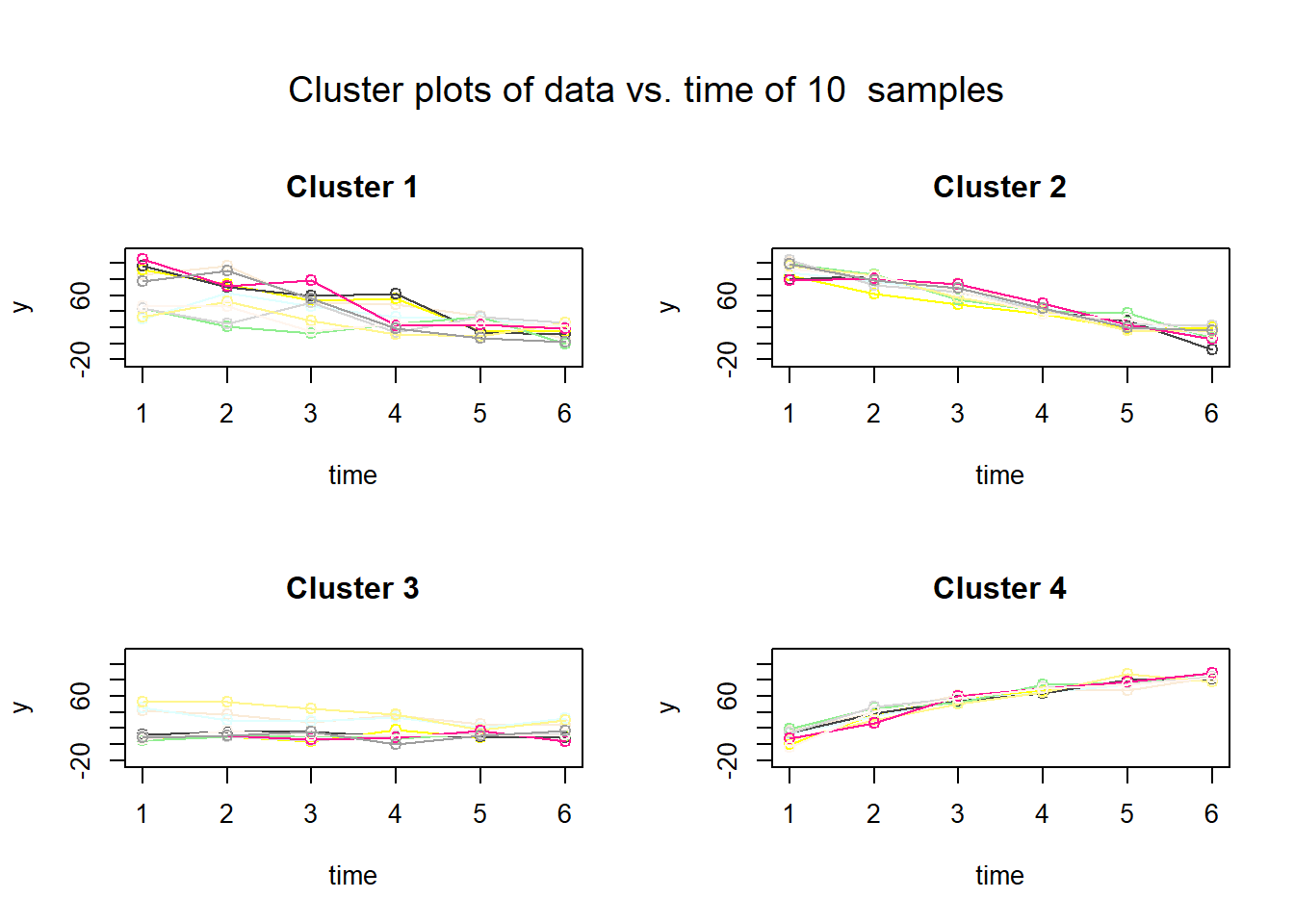This function selects 10 random trajectories from each cluster and plots them using randomly selected colours. The user can specify the number of trajectories to plot, the colours or any other generic plotting parameter. The user can request that trajectories from only one cluster be plotted.

The $$\texttt{plotMeanTraj}$$ function plots the mean trajectory of every cluster. The user can request that trajectories from only one cluster be plotted.

plotMeanTraj(s3)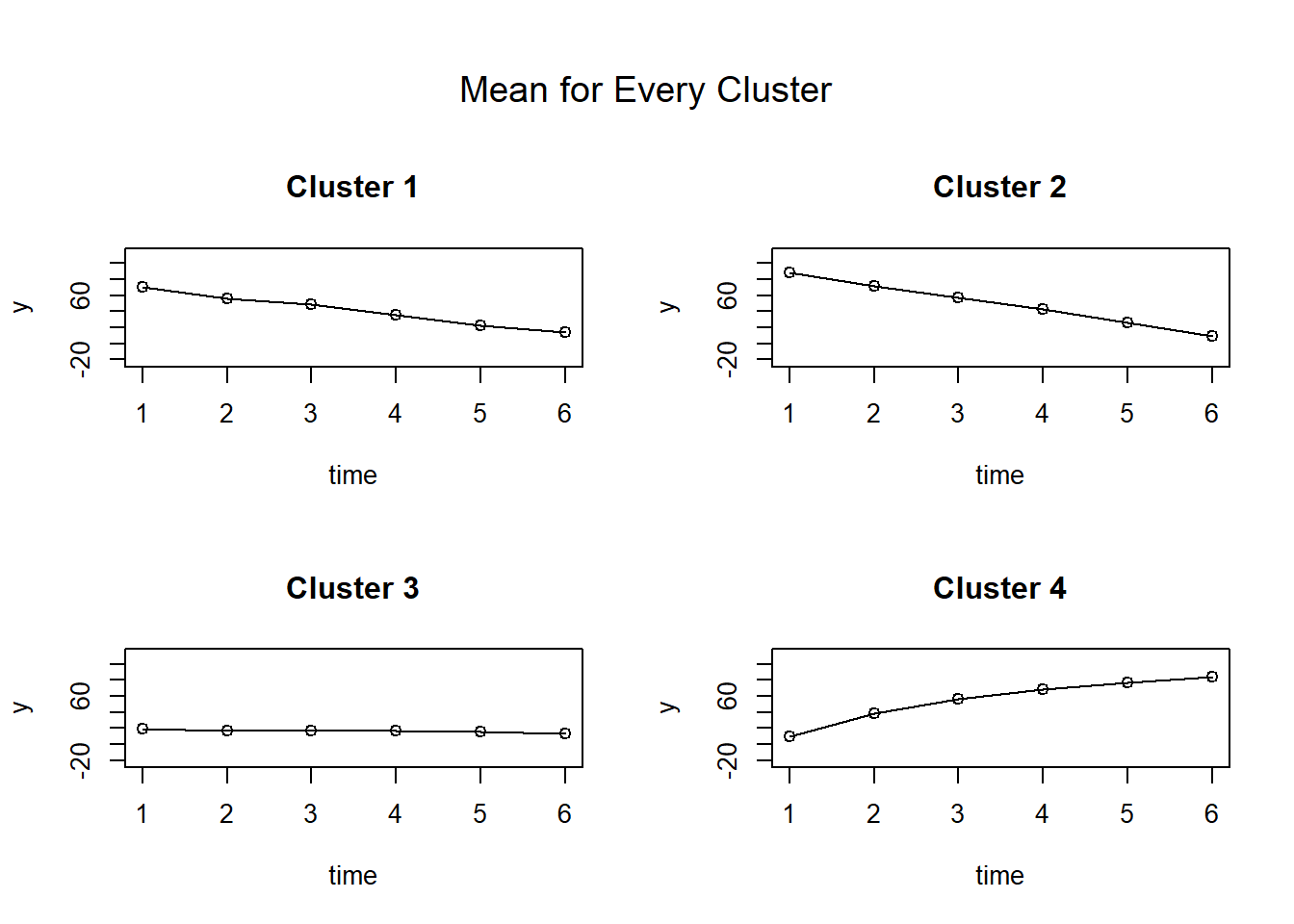The $$\texttt{plotMedTraj}$$ function plots the median trajectory of every cluster with 10th and 90th percentiles. The user can request that trajectories from only one cluster be plotted.

plotMedTraj(s3)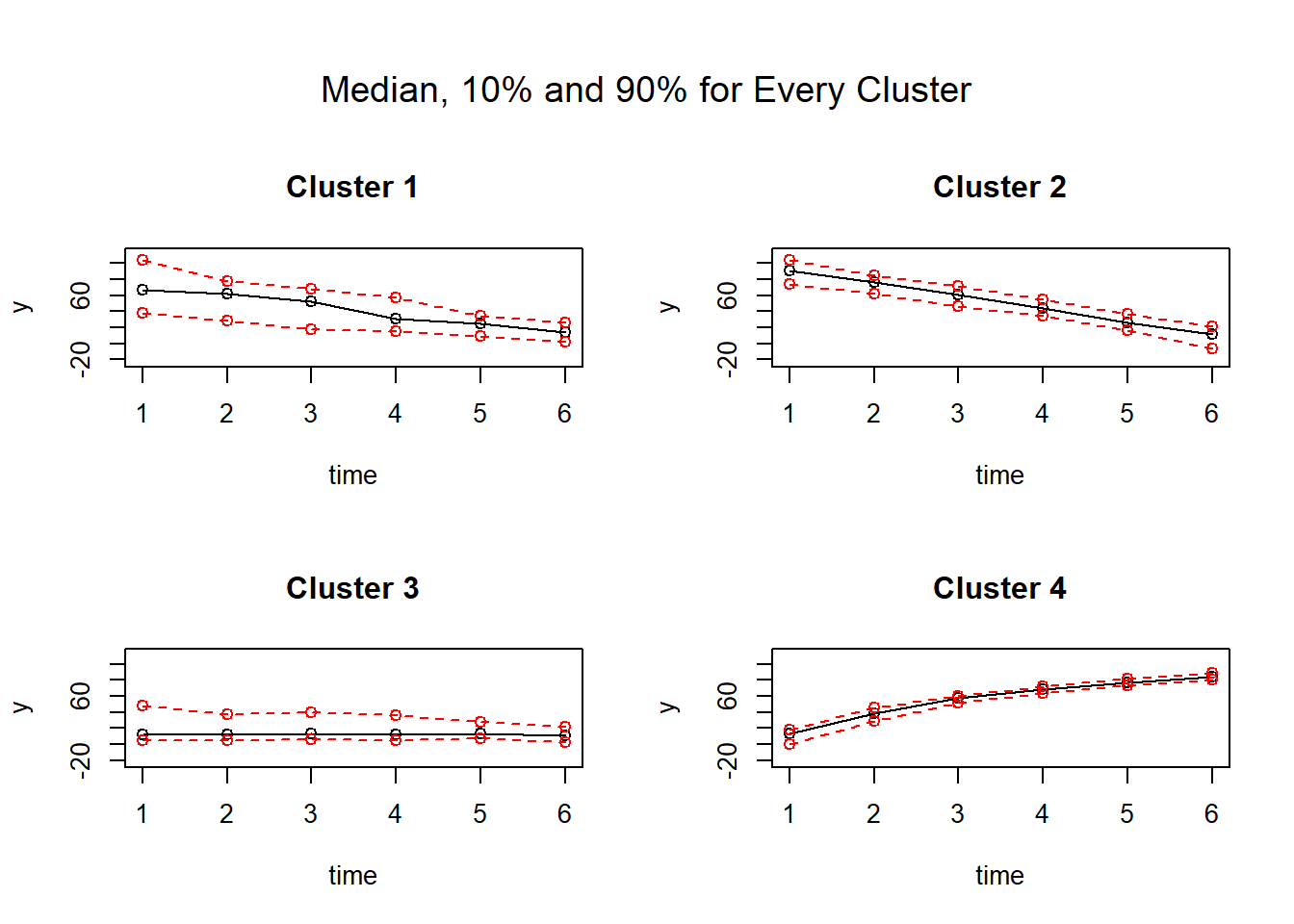The $$\texttt{plotBoxplotTraj}$$ function will plot the box-plot distribution of every time point in each cluster. The user can request that trajectories from only one cluster be plotted.

plotBoxplotTraj(s3)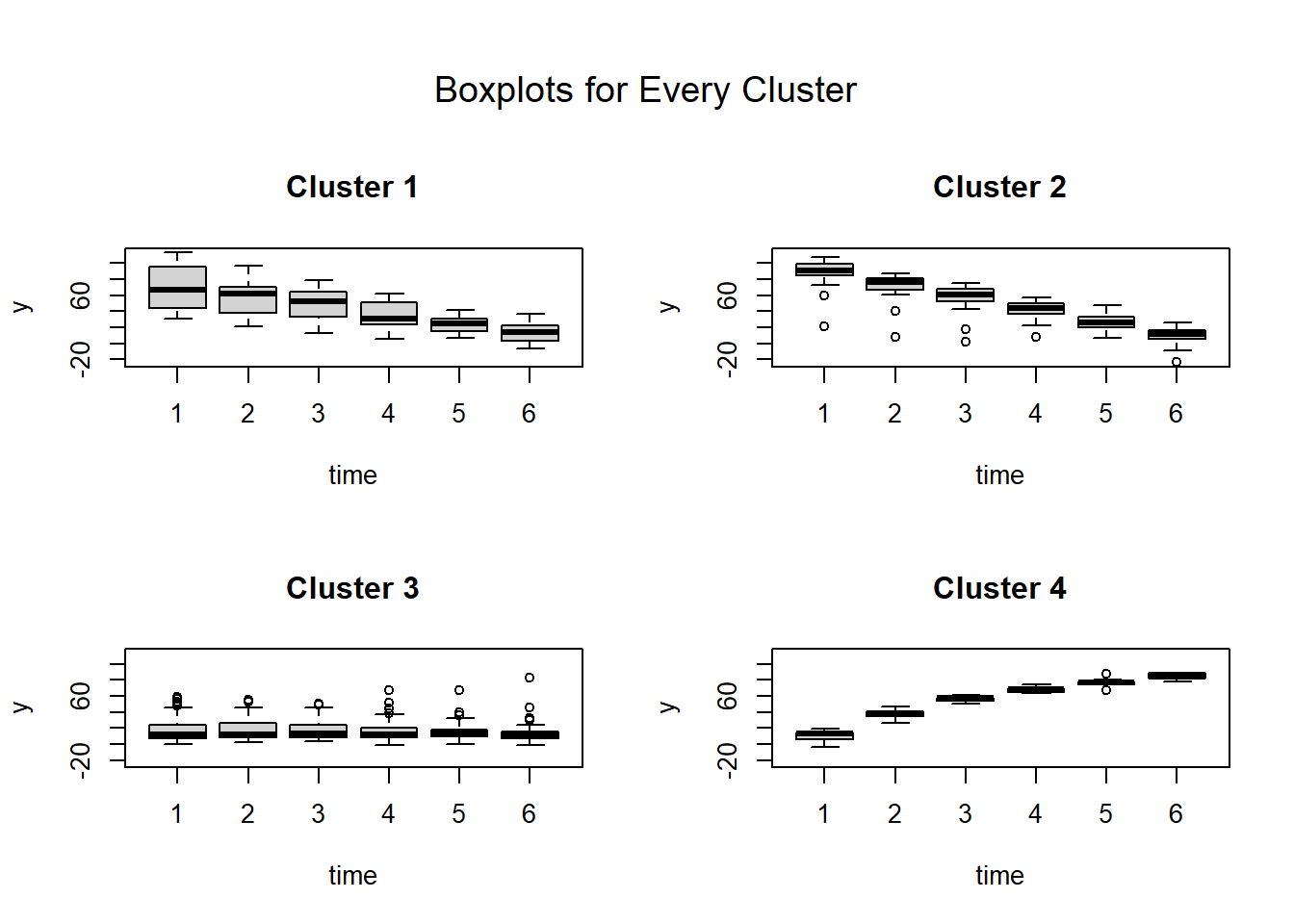The $$\texttt{plotCombTraj}$$ function will plot the mean or median of all the clusters on one single graph. Different colours can be selected as well as different line styles.

plotCombTraj(s3)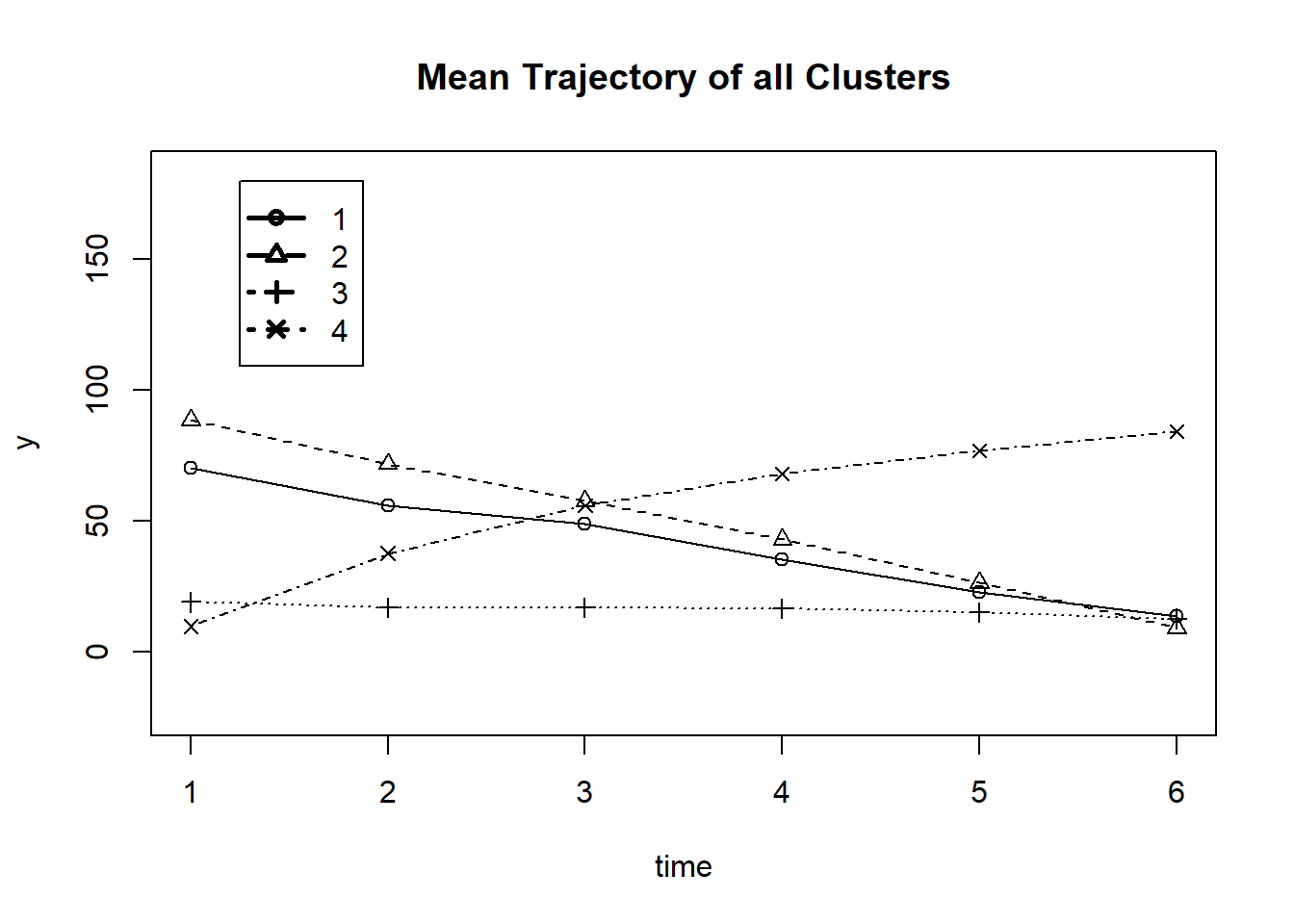1. Department Social and Preventive Medicine, Université de Montréal, CHUM Research Centre↩︎

2. Statistical Programming, CHUM Research Centre↩︎